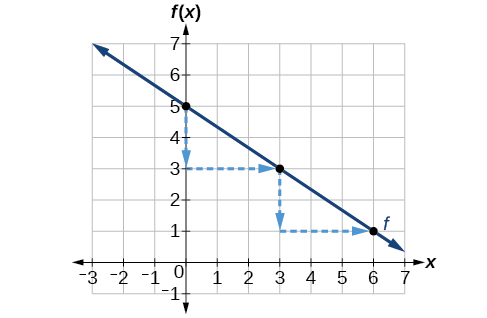# 4.1 Linear functions  (Page 8/27)

 Page 8 / 27

The other characteristic of the linear function is its slope .

Let’s consider the following function.

$f\left(x\right)=\frac{1}{2}x+1$

The slope is $\text{\hspace{0.17em}}\frac{1}{2}.\text{\hspace{0.17em}}$ Because the slope is positive, we know the graph will slant upward from left to right. The y- intercept is the point on the graph when $\text{\hspace{0.17em}}x=0.\text{\hspace{0.17em}}$ The graph crosses the y -axis at $\text{\hspace{0.17em}}\left(0,1\right).\text{\hspace{0.17em}}$ Now we know the slope and the y -intercept. We can begin graphing by plotting the point $\text{\hspace{0.17em}}\left(0,1\right).\text{\hspace{0.17em}}$ We know that the slope is the change in the y -coordinate over the change in the x -coordinate. This is commonly referred to as rise over run, $\text{\hspace{0.17em}}m=\frac{\text{rise}}{\text{run}}.\text{\hspace{0.17em}}$ From our example, we have $\text{\hspace{0.17em}}m=\frac{1}{2},$ which means that the rise is 1 and the run is 2. So starting from our y -intercept $\text{\hspace{0.17em}}\left(0,1\right),$ we can rise 1 and then run 2, or run 2 and then rise 1. We repeat until we have a few points, and then we draw a line through the points as shown in [link] .

## Graphical interpretation of a linear function

In the equation $\text{\hspace{0.17em}}f\left(x\right)=mx+b$

• $b\text{\hspace{0.17em}}$ is the y -intercept of the graph and indicates the point $\text{\hspace{0.17em}}\left(0,b\right)\text{\hspace{0.17em}}$ at which the graph crosses the y -axis.
• $m\text{\hspace{0.17em}}$ is the slope of the line and indicates the vertical displacement (rise) and horizontal displacement (run) between each successive pair of points. Recall the formula for the slope:

Do all linear functions have y -intercepts?

Yes. All linear functions cross the y-axis and therefore have y-intercepts. (Note: A vertical line is parallel to the y-axis does not have a y-intercept, but it is not a function .)

Given the equation for a linear function, graph the function using the y -intercept and slope.

1. Evaluate the function at an input value of zero to find the y- intercept.
2. Identify the slope as the rate of change of the input value.
3. Plot the point represented by the y- intercept.
4. Use $\text{\hspace{0.17em}}\frac{\text{rise}}{\text{run}}\text{\hspace{0.17em}}$ to determine at least two more points on the line.
5. Sketch the line that passes through the points.

## Graphing by using the y- Intercept and slope

Graph $\text{\hspace{0.17em}}f\left(x\right)=-\frac{2}{3}x+5\text{\hspace{0.17em}}$ using the y- intercept and slope.

Evaluate the function at $\text{\hspace{0.17em}}x=0\text{\hspace{0.17em}}$ to find the y- intercept. The output value when $\text{\hspace{0.17em}}x=0\text{\hspace{0.17em}}$ is 5, so the graph will cross the y -axis at $\text{\hspace{0.17em}}\left(0,5\right).$

According to the equation for the function, the slope of the line is $\text{\hspace{0.17em}}-\frac{2}{3}.\text{\hspace{0.17em}}$ This tells us that for each vertical decrease in the “rise” of $\text{\hspace{0.17em}}–2\text{\hspace{0.17em}}$ units, the “run” increases by 3 units in the horizontal direction. We can now graph the function by first plotting the y -intercept on the graph in [link] . From the initial value $\text{\hspace{0.17em}}\left(0,5\right)\text{\hspace{0.17em}}$ we move down 2 units and to the right 3 units. We can extend the line to the left and right by repeating, and then drawing a line through the points.Graph of f ( x ) = −2 / 3 x + 5 and shows how to calculate the rise over run for the slope.

Find a point on the graph we drew in [link] that has a negative x -value.

Possible answers include $\text{\hspace{0.17em}}\left(-3,7\right),\text{\hspace{0.17em}}$ $\left(-6,9\right),\text{\hspace{0.17em}}$ or $\text{\hspace{0.17em}}\left(-9,11\right).$

## Graphing a function using transformations

Another option for graphing is to use a transformation of the identity function $\text{\hspace{0.17em}}f\left(x\right)=x.\text{\hspace{0.17em}}$ A function may be transformed by a shift up, down, left, or right. A function may also be transformed using a reflection, stretch, or compression.

what is the function of sine with respect of cosine , graphically
tangent bruh
Steve
cosx.cos2x.cos4x.cos8x
sinx sin2x is linearly dependent
what is a reciprocal
The reciprocal of a number is 1 divided by a number. eg the reciprocal of 10 is 1/10 which is 0.1
Shemmy
Reciprocal is a pair of numbers that, when multiplied together, equal to 1. Example; the reciprocal of 3 is ⅓, because 3 multiplied by ⅓ is equal to 1
Jeza
each term in a sequence below is five times the previous term what is the eighth term in the sequence
I don't understand how radicals works pls
How look for the general solution of a trig function
stock therom F=(x2+y2) i-2xy J jaha x=a y=o y=b
sinx sin2x is linearly dependent
cr
root under 3-root under 2 by 5 y square
The sum of the first n terms of a certain series is 2^n-1, Show that , this series is Geometric and Find the formula of the n^th
cosA\1+sinA=secA-tanA
Wrong question
why two x + seven is equal to nineteen.
The numbers cannot be combined with the x
Othman
2x + 7 =19
humberto
2x +7=19. 2x=19 - 7 2x=12 x=6
Yvonne
because x is 6
SAIDI
what is the best practice that will address the issue on this topic? anyone who can help me. i'm working on my action research.
simplify each radical by removing as many factors as possible (a) √75
how is infinity bidder from undefined?ByByBy Prateek AshtikarBy Stephen VoronBy Mary MateraBy OpenStaxBy Dindin SecretoBy Darlene PaliswatBy Stephen VoronBy Savannah ParrishBy Dionne MahaffeyBy OpenStax